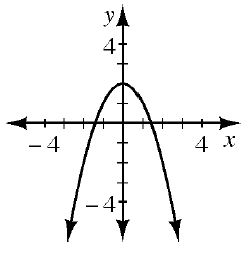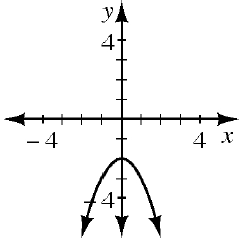### Home > INT2 > Chapter 10 > Lesson 10.2.4 > Problem10-99

10-99.

Match each equation below with its corresponding graph. Can you do this without making any tables? Explain your selections.

1. $y = -x^2 - 2$

1.1. $y = x^2 - 2$

1.1. $y = -x^2 + 2$

1.Determine the direction of the opening and $y$-intercept for each of the given equations.

$2$